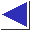# comp.lang.c FAQ list ·Question 4.8

Q: I have a function which accepts, and is supposed to initialize, a pointer:

```	void f(int *ip)
{
static int dummy = 5;
ip = &dummy;
}
```
But when I call it like this:
```	int *ip;
f(ip);
```
the pointer in the caller remains unchanged.

A: Are you sure the function initialized what you thought it did? Remember that arguments in C are passed by value. In the code above, the called function alters only the passed copy of the pointer. To make it work as you expect, one fix is to pass the address of the pointer (the function ends up accepting a pointer-to-a-pointer; in this case, we're essentially simulating pass by reference):

```	void f(ipp)
int **ipp;
{
static int dummy = 5;
*ipp = &dummy;
}

...

int *ip;
f(&ip);
```

Another solution is to have the function return the pointer:

```	int *f()
{
static int dummy = 5;
return &dummy;
}

...

int *ip = f();
```